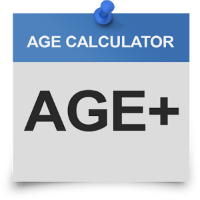## SAT Math Lesson-10

SAT Math Lesson-10 Word Problems (Venn Diagram) In matriculation/O-Level mathematics, you’ve studied overlapping sets or Venn diagram. This topic also tested in exams like this. So let’s start with two variable Venn diagram. Suppose, In a class of 100 students, 70 students study Math and 80 students study English. How Read More …

## SAT Math Lesson-09

SAT Math Lesson-09 Word Problems (Speed, Distance & Time) Complex Scenarios: It’s time to start absorbing the complex concepts in the same topic that you’ve learned in yesterday lecture. Train Scenarios: One Train Scenario: Let’s take train scenario, where the length of the train also come into calculation. For instance Read More …

## SAT Math Lesson-08

SAT Math Lesson-08 Word Problems (Speed, Distance & Time) As most of the students are familiar with the equation used in physics, if you ever read, that gives relationship between Distance, Speed and Time. The more the speed, the lesser is the time it take to cover some distance and Read More …

## SAT Math Lesson-07

SAT Math Lesson-07 Word Problems (Work-Rate) Let’s discuss hard difficulty level scenarios of Work-Rate problem at this stage: A wider pipe can fill 2.5 times faster than a narrow pipe. Working together, both pipes can fill the pool in 2.5 hours. How long will it take to fill the pool Read More …

## SAT Math Lesson-06

SAT Math Lesson-06 Word Problems (Work-Rate) As we read before in ‘GAT Quantitative Part-1’, the relationship between Distance, Speed & Time. Distance is like Work done (that how much distance covered means how much work done). Speed is like Rate (that how much miles in a hour the work takes Read More …

## SAT Math Lesson-05

SAT Math Lesson-05 Word Problems (Fractions & Percents) Let’s take another scenario where percentage change formula is used, If x is 60% greater than y, then y is what percent less than x? Solution: As x is 60% greater than y ⇒ x = y (1 + 0.60) = 1.6y Read More …

## SAT Math Lesson-04

SAT Math Lesson-04 Word Problems (Fractions & Percents) Fractions & Percents are one of the most common type of word problems that always come in such exams. The reason of being important is that many professionals have to face such problems in their daily routine while dealing with clients or Read More …

## SAT Math Lesson-03

SAT Math Lesson-03 Word Problems (Ratios & Proportions) Simple concepts & scenarios: There are two types of ratios namely: 1). Simple ratios, 2). Compound ratios   1). Simple ratios: Simple ratios involves two variables. For instance, let’s suppose Boys to Girls ratio in a school is 4:3. There are two Read More …

## SAT Math Lesson-02

SAT Math Lesson-02 Word Problems (Mixtures) Mixtures are one of the most toughest part of word problems that many students feel uncomfortable when they see such questions during their exam. And worst part is, it usually come with mixture of different topics like percent, fractions, and ratios etc. Let’s discuss Read More …

## SAT Math Lesson-01SAT Math Lesson-01 Word Problems (Age) If you ask me which topic is the most easiest in the whole quantitative section, the answer is ‘Age’. And you’ll be witness after covering this topic here using only one method and solving every question of any difficulty level that come from ‘Age’ Read More …